# Thermodynamics Chapter 20 Thermodynamics Prediction of whether change

• Slides: 30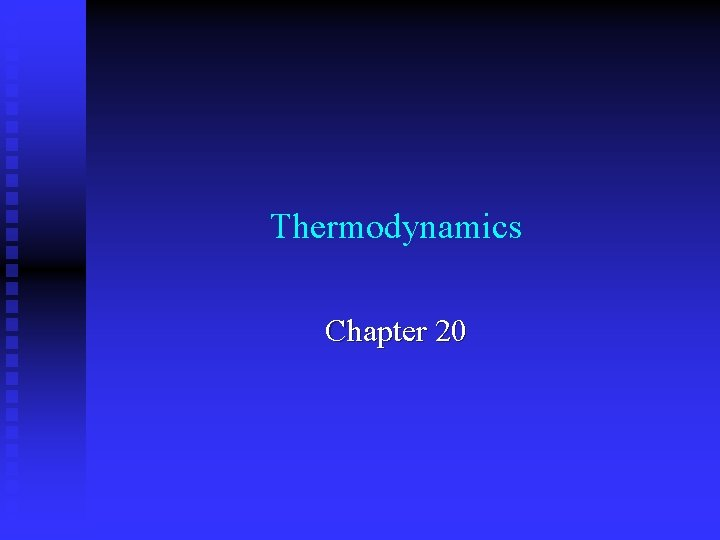Thermodynamics Chapter 20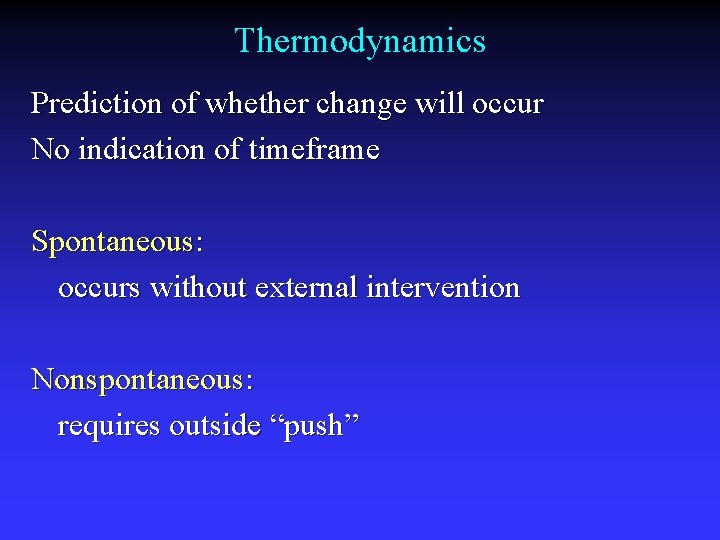Thermodynamics Prediction of whether change will occur No indication of timeframe Spontaneous: occurs without external intervention Nonspontaneous: requires outside “push”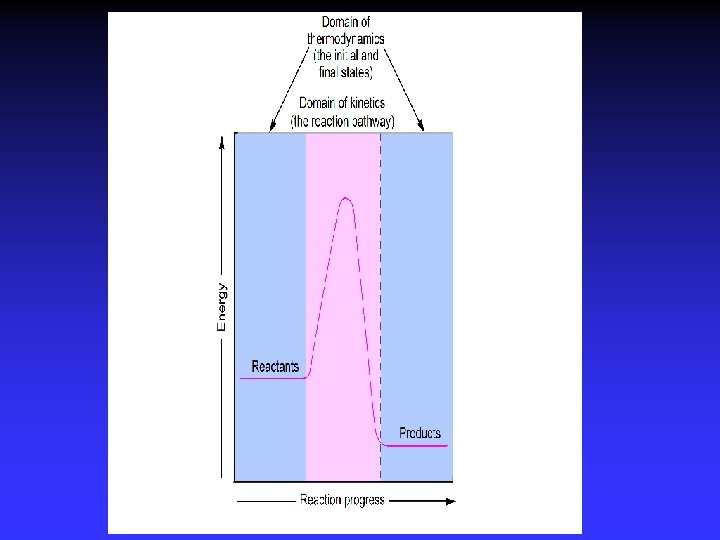Entropy and Spontaneity Driving force for a spontaneous change is an increase in entropy of the universe Entropy, S: measure of disorder Spontaneous change implies: more order less order fewer ways of arranging particles more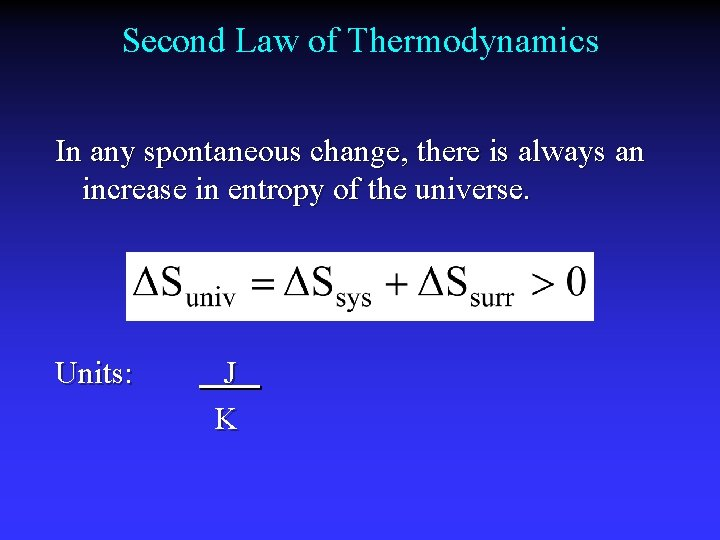Second Law of Thermodynamics In any spontaneous change, there is always an increase in entropy of the universe. Units: J KEntropy 1877 Ludwig Boltzmann: k = Boltzmann constant, R/NA W = no. of possible arrangements Third Law of Thermodynamics: The entropy of a perfect crystal at 0 K is zero.Positional Entropy Why does a gas expand into a vacuum? Expanded state has highest positional probability of states available.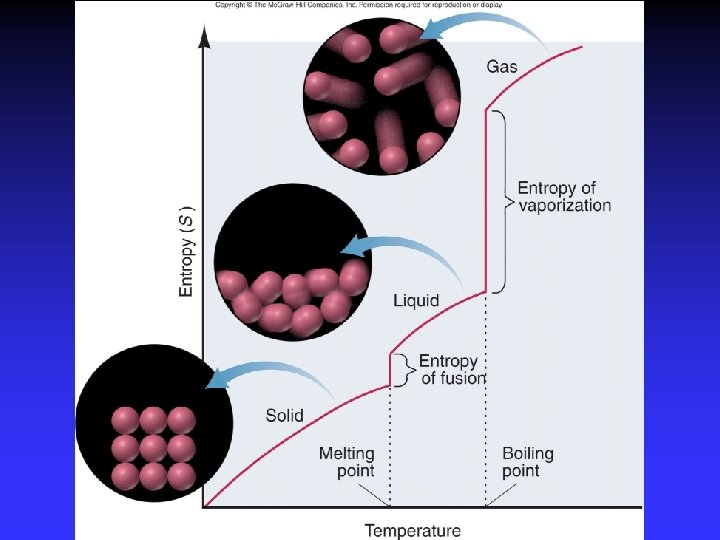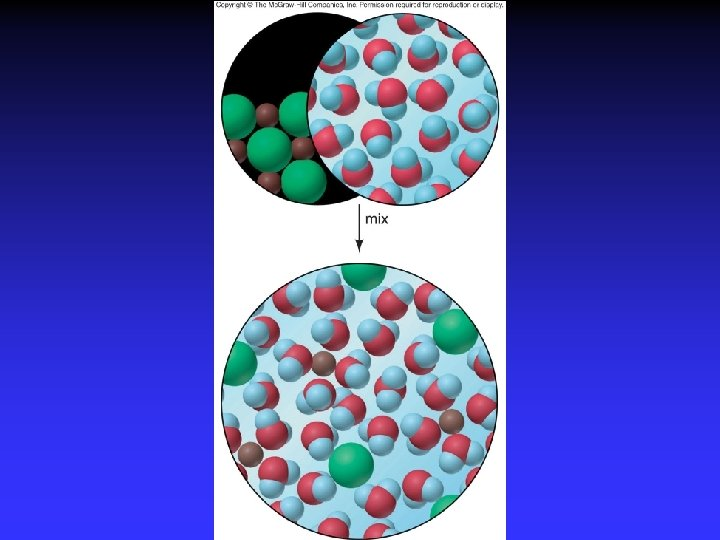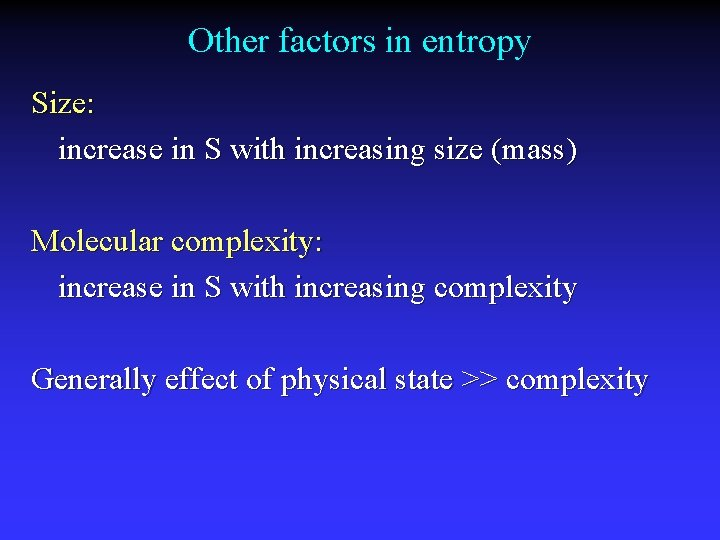Other factors in entropy Size: increase in S with increasing size (mass) Molecular complexity: increase in S with increasing complexity Generally effect of physical state >> complexity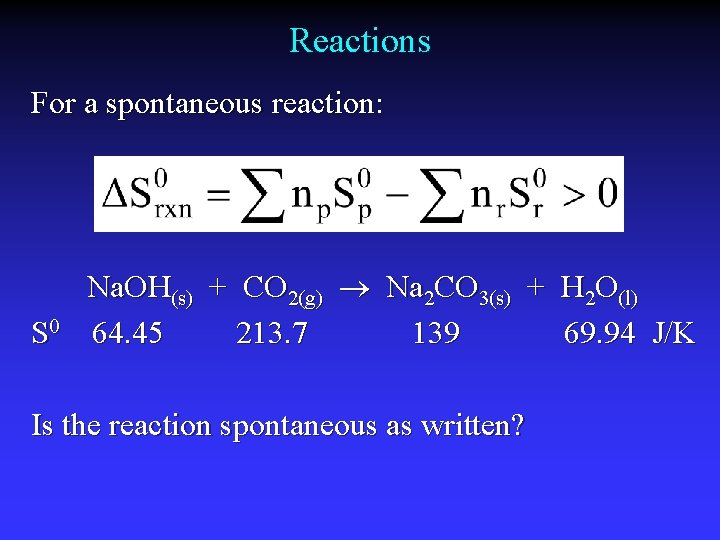Reactions For a spontaneous reaction: S 0 Na. OH(s) + CO 2(g) Na 2 CO 3(s) + H 2 O(l) 64. 45 213. 7 139 69. 94 J/K Is the reaction spontaneous as written?Spontaneity and S Spontaneous: Nonspontaneous: At equilibrium: Suniv > 0 Suniv < 0 Suniv = 0 Ssys can be positive if Ssurr increases enoughSurroundings and Suniv Surroundings add or remove heat Exothermic: qsys qsurr < > 0 0 so Ssurr > 0 Endothermic: qsys > 0 qsurr < 0 so Ssurr < 0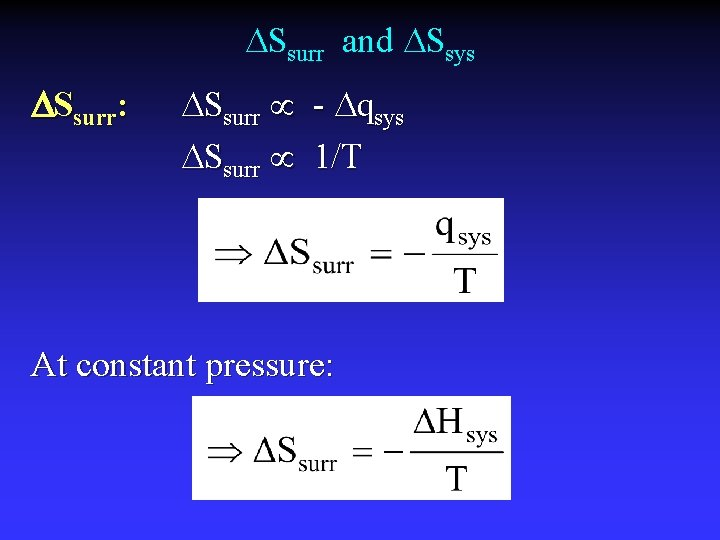Ssurr and Ssys Ssurr: Ssurr - qsys Ssurr 1/T At constant pressure:The Math Given: @constant P: Multiply by T: Result:Reactions and G G 0: Standard Free Energy Reactants in standard states are converted to products in standard statesGibb’s Free Energy Overall criterion for spontaneity from the standpoint of the system A process at constant temp. and pressure is spontaneous in the direction G decreasesG = H - T S H S G Spontaneous? “Good”: H < 0 “Good”: S > 0 “Good”: G < 0 - + - - - ? + + ? + - + At all temperatures At low temperatures At high temperatures Not at any temperature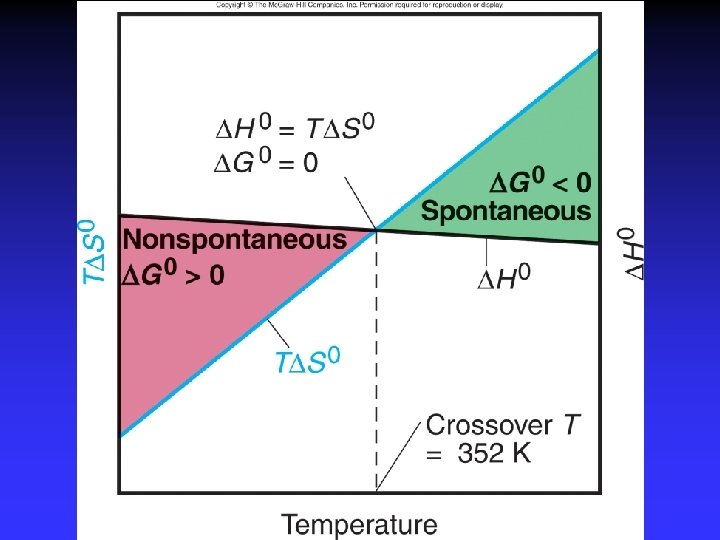Summary G < 0 Spontaneous as written G > 0 Not spontaneous as written Reverse process spontaneous G = 0 At equilibrium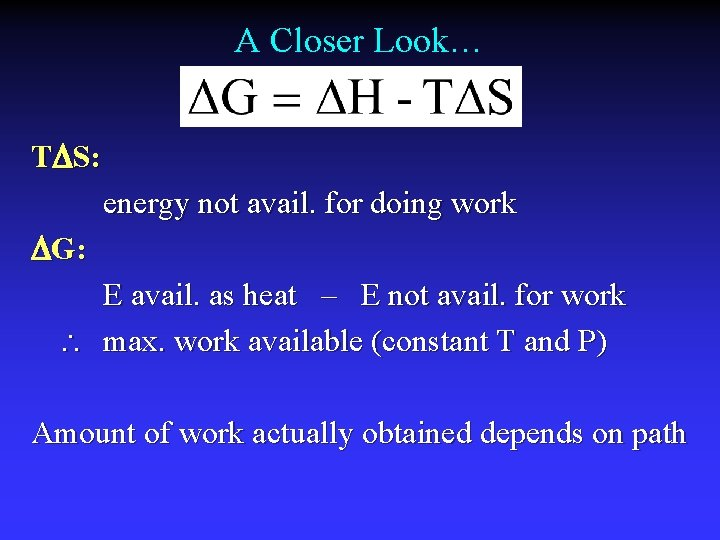A Closer Look… T S: energy not avail. for doing work G: E avail. as heat – E not avail. for work max. work available (constant T and P) Amount of work actually obtained depends on pathG and Work G Spontaneous Nonspontaneous max. work obtainable min. work required Work and path-dependence wmax (wmin) process performed reversibly theoretical wactual < wmax performed irreversibly real world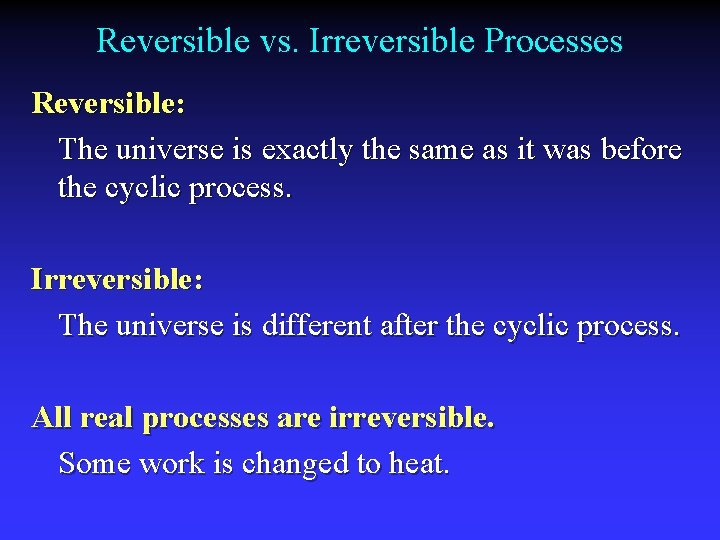Reversible vs. Irreversible Processes Reversible: The universe is exactly the same as it was before the cyclic process. Irreversible: The universe is different after the cyclic process. All real processes are irreversible. Some work is changed to heat.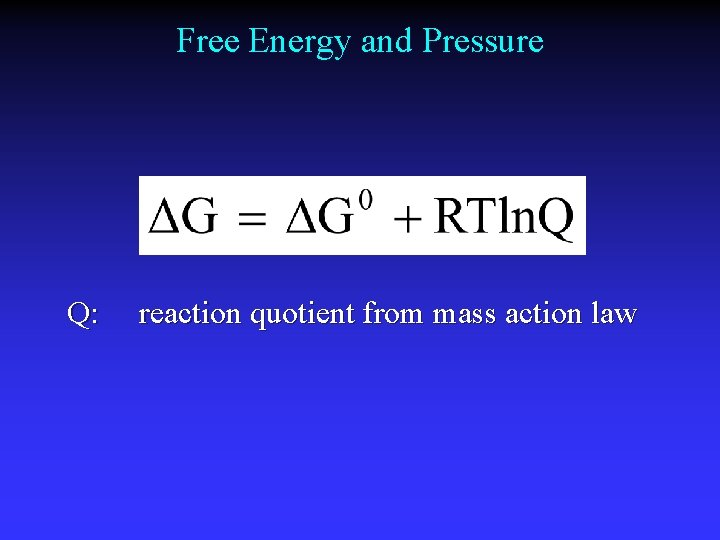Free Energy and Pressure Q: reaction quotient from mass action law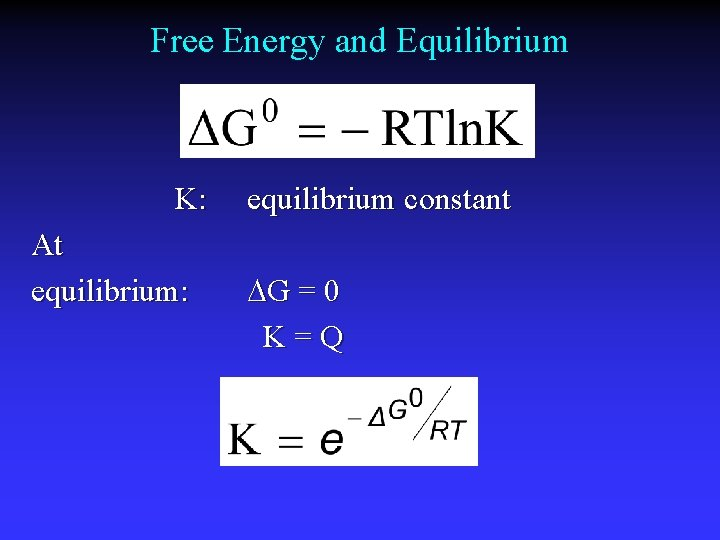Free Energy and Equilibrium K: At equilibrium: equilibrium constant G = 0 K=Q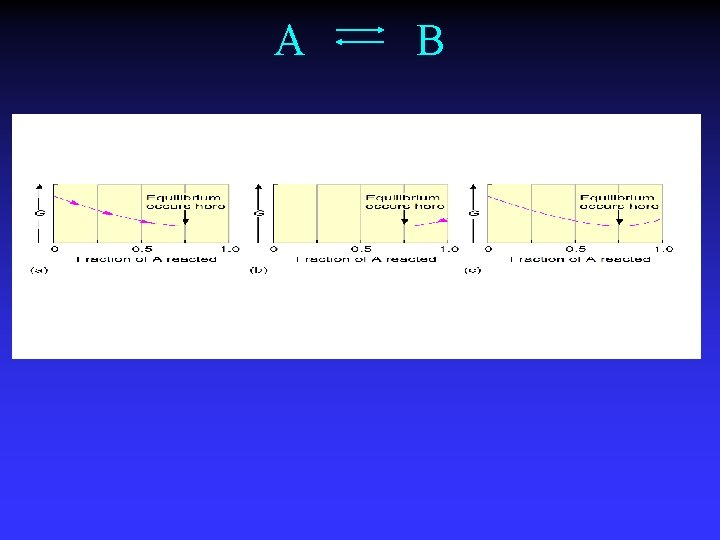A B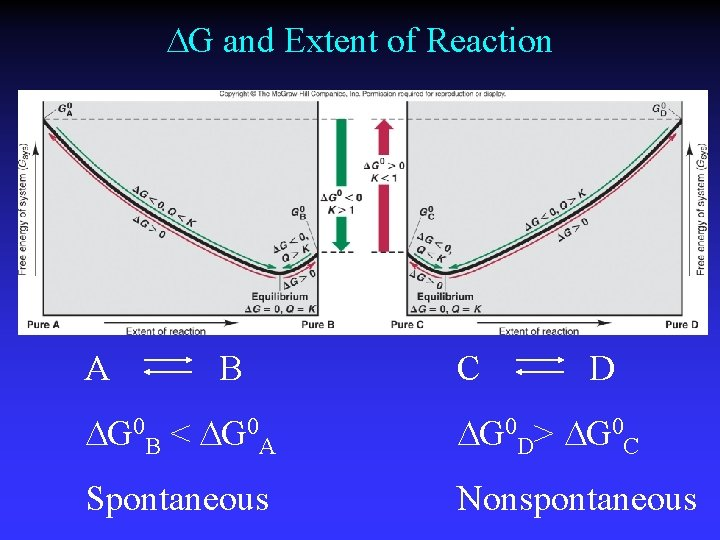G and Extent of Reaction A B C D G 0 B < G 0 A G 0 D> G 0 C Spontaneous Nonspontaneous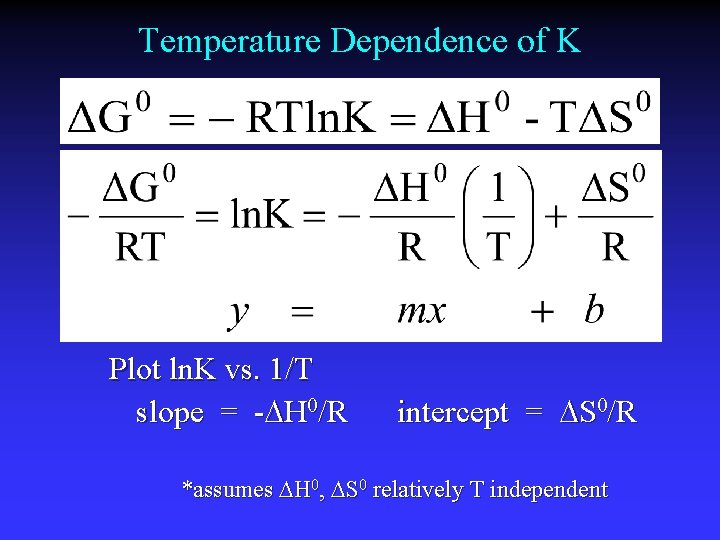Temperature Dependence of K Plot ln. K vs. 1/T slope = - H 0/R intercept = S 0/R *assumes H 0, S 0 relatively T independent